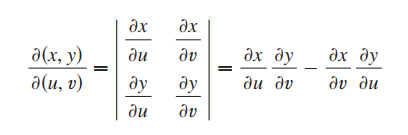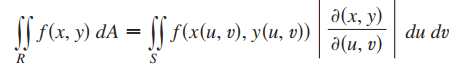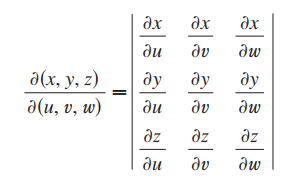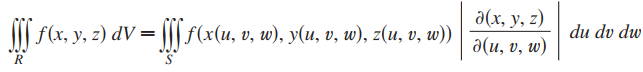Need Help?

Subscribe to Calculus C

###### \${selected_topic_name}
• Notes
• The Jacobian of the transformation T given by x =g(u, v) and y=h(u, v)  is• Change of Variables in a Double Integral• The Jacobian of T is the following 3 X 3 determinant:• Change of Variables in a triple Integral: